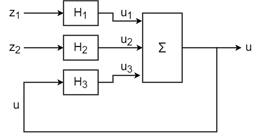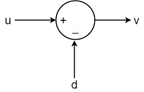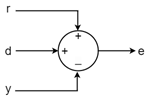# sumblk

Summing junction for name-based interconnections

## Syntax

``S = sumblk(formula)``
``S = sumblk(formula,signalsize)``
``S = sumblk(formula,signames1,...,signamesN)``

## Description

example

``` `S = sumblk(formula)` creates the summing junction described by `formula` in the form of a transfer function (`tf`) model, `S`. The string `formula` specifies an equation that relates the scalar input and output signals of `S`, such as `"e = r - y"`. The function sets the `InputName` and `OutputName` properties of `S` based on `formula`. Use `S` in conjunction with `connect` to interconnect dynamic system models and derive aggregate models for block diagrams. ```

example

````S = sumblk(formula,signalsize)` returns a vector-valued summing junction. The input and output signals are vectors with `signalsize` elements. `sumblk` sets the `InputName` and `OutputName` properties by vector expansion of the signal names in `formula`.```

example

````S = sumblk(formula,signames1,...,signamesN)` replaces placeholders in `formula` by the signal names `signames`. Indicate a placeholder in `formula` by using a signal name beginning with `%` . The number of `signames` arguments must match the number of placeholders in `formula`. The first placeholder in `formula` is replaced by `signames`1, the second by `signames`2, and so on. ```

## Examples

collapse all

Create the summing junction of the following illustration. All signals are scalar-valued.This summing junction has the formula `u = u1 + u2 + u3`. Use this formula with `sumblk` to create the summing junction.

`S = sumblk('u = u1 + u2 + u3');`

`S` is the transfer function (`tf`) representation of the sum `u = u1 + u2 + u3`. That is, `S` is a static-gain `tf` with three inputs and one output, which is equal to the sum of the inputs. The transfer function `S` gets its input and output names from the formula.

`S.OutputName`
```ans = 1x1 cell array {'u'} ```
`S.InputName`
```ans = 3x1 cell {'u1'} {'u2'} {'u3'} ```

Therefore, you can use `S` with the name-based syntax of the `connect` command to build aggregate models such as the system of the following block diagram.To do so, create LTI models for `H1`, `H2`, and `H3`, and name their inputs and outputs according to the diagram. For this example, use transfer functions.

```H1 = tf(1,[1 2],"InputName","z1","OutputName","u1"); H2 = tf([1 -2],[1 1],"InputName","z2","OutputName","u2"); H3 = tf(2,[2 1],"InputName","u","OutputName","u3");```

Connect the models and the summing junction to create an aggregate model with inputs `z1` and `z2` and output `u`. The `connect` command automatically connects the outputs of the components to inputs with matching names.

```T = connect(H1,H2,H3,S,{"z1","z2"},{"u"}); T.InputName```
```ans = 2x1 cell {'z1'} {'z2'} ```
`T.OutputName`
```ans = 1x1 cell array {'u'} ```

Create the summing junction `v = u - d` where `u`, `d`, `v` are vector-valued signals of length 2. This summing junction is shown in the following diagram, where each arrow represents two signals.This summing junction outputs `v(1) = u(1) - d(1)` and `v(2) = u(2) - d(2)`. To create this junction using `sumblk`, specify both the formula and the signal length.

```S = sumblk('v = u - d',2); size(S)```
```Transfer function with 2 outputs and 4 inputs. ```

The result is a transfer function with four inputs and two outputs: two inputs each for `d` and `u`, and two outputs for `v`. `sumblk` automatically performs vector expansion of the signal names and assigns them to `S.InputName` and `S.OutputName`.

`S.InputName`
```ans = 4x1 cell {'u(1)'} {'u(2)'} {'d(1)'} {'d(2)'} ```
`S.OutputName`
```ans = 2x1 cell {'v(1)'} {'v(2)'} ```

You can connect `S` to other dynamic system models to build aggregate models, as you would use any other MIMO transfer function. For instance, suppose that you want to create a model representing the following two-channel feedback loop, that is, a feedback loop in which each line in the diagram represents two signals.Create two-input, two-output models for `H1` and `H2`, and set their `InputName` and `OutputName` properties as indicated by the diagram. For this example, use random state-space models.

```H1 = rss(3,2,2); H1.InputName = 'v'; H1.OutputName = 'w'; H2 = rss(3,2,2); H2.InputName = 'w'; H2.OutputName = 'd';```

Note that the signal names in the dynamic system models are automatically expanded, just as `sumblk` expands the signal names of `S`. For instance, examine the outputs of `H1`.

`H1.OutputName `
```ans = 2x1 cell {'w(1)'} {'w(2)'} ```

The `connect` command also performs this expansion. Thus, you can assemble the aggregate system with the following command.

```T = connect(S,H1,H2,'u','w'); size(T)```
```State-space model with 2 outputs, 2 inputs, and 6 states. ```

As shown in the example Summing Junction with Vector-Valued Signals, when you create a summing junction for vector signals, by default `sumblk` appends an index to the signal names that you provide. If you need to specify distinct signal names instead of this vector expansion, use a placeholder in the summing-junction formula to represent the signals you want to name. Then, use the `signames` input argument to provide the specific names to substitute for the placeholder in creating the summing junction.

For instance, create a summing junction having the following formula.

`$\begin{array}{l}e\left(1\right)=setpoint\left(1\right)-alpha\\ e\left(2\right)=setpoint\left(2\right)-q\end{array}$`

This formula is the summing junction shown in the following diagram, where `setpoint` represents two inputs and `e` represents two outputs.To create this summing junction, allow `sumblk` to expand `e` and `setpoint`, but use a signal name beginning with `%` as a placeholder for the specific signal names you provide in the `signames` argument.

```formula = 'e = setpoint - %y'; signames = ["alpha","q"]; S = sumblk(formula,signames);```

`sumblk` replaces the placeholder `%y` with the signal names `alpha` and `q` and expands the other signal names. Note that `sumblk` takes the size of the vector signals from the number of signals in `signames`. Thus, the `setpoint` input and `e` output of `S` each contain two signals.

`S.InputName`
```ans = 4x1 cell {'setpoint(1)'} {'setpoint(2)'} {'alpha' } {'q' } ```
`S.OutputName`
```ans = 2x1 cell {'e(1)'} {'e(2)'} ```

This syntax is particularly useful when you want to create a summing junction to connect existing models whose `InputName` or `OutputName` properties are already set. For instance, consider the following two-input, two-output state-space model.

```A = [-0.004 0.03; 0.03 -0.19]; B = [0.3 0.2; -0.06 0]; C = [0.99 0.5; 0 0]; D = 0; G = ss(A,B,C,D); G.InputName = {'angle','rate'}; G.OutputName = {'current','temp'};```

Create a summing junction that subtracts the outputs of `G` from a pair of reference inputs, such that

`$\begin{array}{l}e\left(1\right)=ref\left(1\right)-current\\ e\left(2\right)=ref\left(2\right)-temp\end{array}$`

To do so, use a placeholder for these signals in the formula. Then, use `G.OutputName` as the `signames` input.

```S = sumblk('e = ref - %outputs',G.OutputName); S.InputName```
```ans = 4x1 cell {'ref(1)' } {'ref(2)' } {'current'} {'temp' } ```

You can use multiple placeholders to replace multiple elements of the formula. `sumblk` replaces the placeholders in the order you provide them. For instance, give the reference signals specific names instead of `ref(1)` and `ref(2)`.

```refnames = ["refcur","reftemp"]; S = sumblk('e = %refs - %outputs',refnames,G.OutputName); S.InputName```
```ans = 4x1 cell {'refcur' } {'reftemp'} {'current'} {'temp' } ```

## Input Arguments

collapse all

Equation that relates the input and output signals of the summing junction transfer function `S`, specified as a string or character vector. For example, consider the summing junction of the following diagram.To represent this summing junction as a three-input, one-output transfer function, use the following commands.

```formula = "e = r + d - y"; S = sumblk(formula);```

For vector-valued signals, you can optionally use one or more placeholders in `formula` to control the names that `sumblk` assigns to `S.InputName` and `S.OutputName`. To put a placeholder in `formula`, use a signal name that begins with `%`. When assigning signal names to `S`, the `sumblk` command replaces each placeholder with the names you provide in the `signames` argument.

Such placeholders are particularly useful for creating summing junctions for connecting existing models that have named signals. For example, if `C` and `G` are dynamic system models with nonempty `InputName` and `OutputName` properties, respectively, you can create a summing junction using the following expression.

`S = sumblk("%e = r - %y",C.InputName,G.OutputName)`

`sumblk` uses the values of `C.InputName` and `G.OutputName` in place of `%e` and `%y`, respectively. The vector dimension of `C.InputName` and `G.OutputName` must match. `sumblk` assigns the signal `r` the same dimension. For an example, see Specify Individual Names in Summing Junction with Vector-Valued Signals.

Number of elements in each input and output signal of `S`, specified as a positive integer. Setting `signalsize` greater than 1 lets you specify a summing junction that operates on vector-valued signals. For instance, `S = sumblk("e = r - y",2)` creates a summing junction where each of the inputs `r` and `y` and the output `e` is a vector signal with two elements. See Summing Junction with Vector-Valued Signals.

Substitute signal names for a placeholder (signal name beginning with `%`) in the argument `formula`, specified as a string array or a cell array of character vectors. Provide one `signames` argument for each placeholder in `formula`.

Specify `signames` as:

• A string array, such as `["alpha","q"]`.

• A cell array of signal names, such as `{'alpha','q'}`.

• The `InputName` or `OutputName` property of a model in the MATLAB® workspace. For example:

`S = sumblk("%e = r - y",C.InputName)`

This command creates a summing junction whose outputs have the same name as the inputs of the model `C` in the MATLAB workspace. Specify Individual Names in Summing Junction with Vector-Valued Signals.

If you use placeholders and the `signames` argument, then `sumblk` sets the vector length of the signals of `S`. If you use multiple placeholders, than all `signames` arguments must have the same number of signals.

## Output Arguments

collapse all

Transfer function for the summing junction, returned as a `tf` model.

`sumblk` sets the `InputName` and `OutputName` properties of `S` using the signal names in `formula`. For instance, if you enter ```S = sumblk("e = r + d - y")```, then `sumblk` creates `S` with `S.InputName = {'r';'d';'y'}` and `S.OutputName = {'e'}`. Use these input and output names to interconnect `S` with other dynamic system models to build composite systems. For an example, see Summing Junction with Scalar-Valued Signals.

If `signalsize` is greater than 1, then `S` has `signalsize` inputs (or outputs) per signal name in `formula`. The function sets `S.InputName` and `S.OutputName` by applying vector expansion to the signal name specified in `formula`, such as `{'e(1)','e(2)',...}`. For an example, see Summing Junction with Vector-Valued Signals.

If you use placeholders in `formula`, then `sumblk` sets the `InputName` and `OutputName` properties of `S` using the names you provide in `signames`. For an example, see Specify Individual Names in Summing Junction with Vector-Valued Signals.

## Version History

Introduced in R2008a